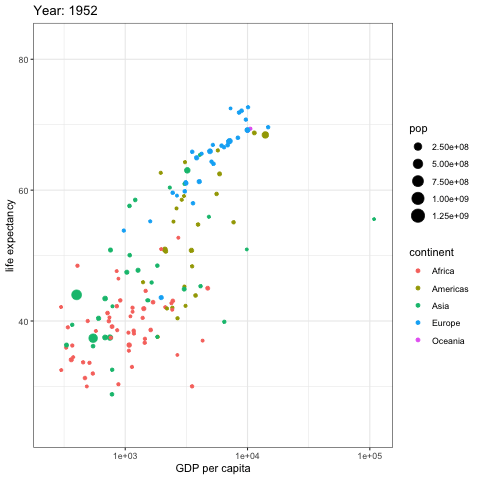# Animated bubble chart with R and gganimate

The `gganimate` package allows to build `animated` chart using the `ggplot2` syntax directly from R. This post shows how to apply it on a bubble chart, to show an evolution in time.

# Animated bubble chart

Before trying to build an animated plot with `gganimate`, make sure you understood how to build a basic bubble chart with R and `ggplot2`.

The idea is to add an additional aesthetics called `transition_..()` that provides a frame variable. For each value of the variable, a step on the chart will be drawn. Here, `transition_time()` is used since the frame variable is numeric.

Note that the `gganimate` automatically performs a transition between state. Several options are available, set using the `ease_aes()` function.``````# Get data:
library(gapminder)

# Charge libraries:
library(ggplot2)
library(gganimate)

# Make a ggplot, but add frame=year: one image per year
ggplot(gapminder, aes(gdpPercap, lifeExp, size = pop, color = continent)) +
geom_point() +
scale_x_log10() +
theme_bw() +
# gganimate specific bits:
labs(title = 'Year: {frame_time}', x = 'GDP per capita', y = 'life expectancy') +
transition_time(year) +
ease_aes('linear')

# Save at gif:
anim_save("271-ggplot2-animated-gif-chart-with-gganimate1.gif")``````

# Use small multiple

Since `gganimate` is a ggplot2 extension, any ggplot2 option can be used to customize the chart. Here, an example using `facet_wrap()` to use small multiple on the previous chart, spliting the chart window per continent.

Important note: this example comes from the gganimate homepage.``````# Get data:
library(gapminder)

# Charge libraries:
library(ggplot2)
library(gganimate)

# Make a ggplot, but add frame=year: one image per year
ggplot(gapminder, aes(gdpPercap, lifeExp, size = pop, colour = country)) +
geom_point(alpha = 0.7, show.legend = FALSE) +
scale_colour_manual(values = country_colors) +
scale_size(range = c(2, 12)) +
scale_x_log10() +
facet_wrap(~continent) +
# Here comes the gganimate specific bits
labs(title = 'Year: {frame_time}', x = 'GDP per capita', y = 'life expectancy') +
transition_time(year) +
ease_aes('linear')

# Save at gif:
anim_save("271-ggplot2-animated-gif-chart-with-gganimate2.gif")``````

Related chart types

## Contact

This document is a work by Yan Holtz. Any feedback is highly encouraged. You can fill an issue on Github, drop me a message on Twitter, or send an email pasting yan.holtz.data with gmail.com.